# RS Aggarwal Solutions for Class 8 Maths Chapter 10 - Profit and Loss Exercise 10D

Students can download RS Aggarwal Solutions for Class 8 Maths Chapter 10- Exercise 10D, Profit and Loss from the links provided below. Our experts have solved the RS Aggarwal Solutions to help students secure good marks in their exams. In Exercise 10D of RS Aggarwal Class 8 Maths, we shall brief all the topics covered in Chapter 10.

## Download PDF of RS Aggarwal Solutions for Class 8 Maths Chapter 10- Profit and Loss Exercise 10D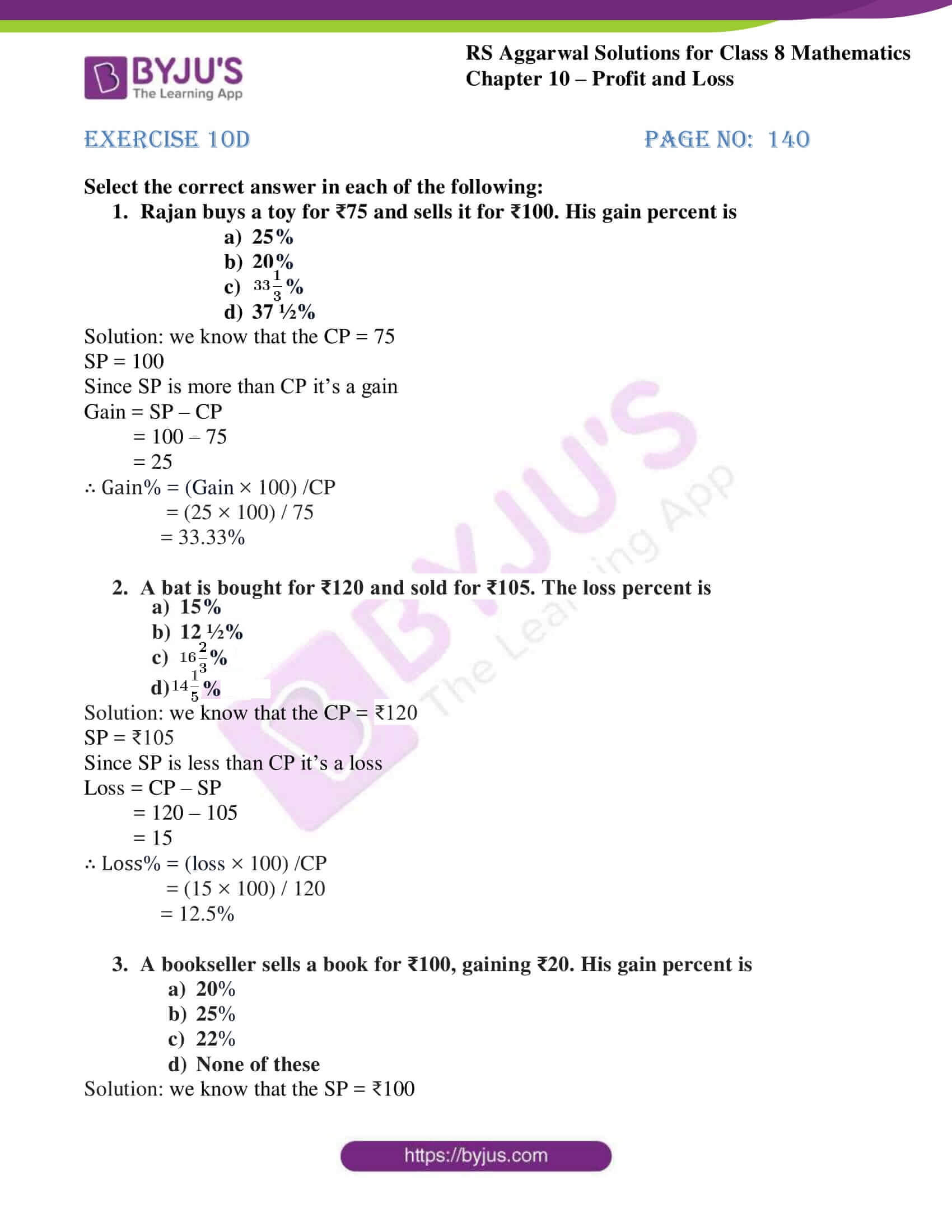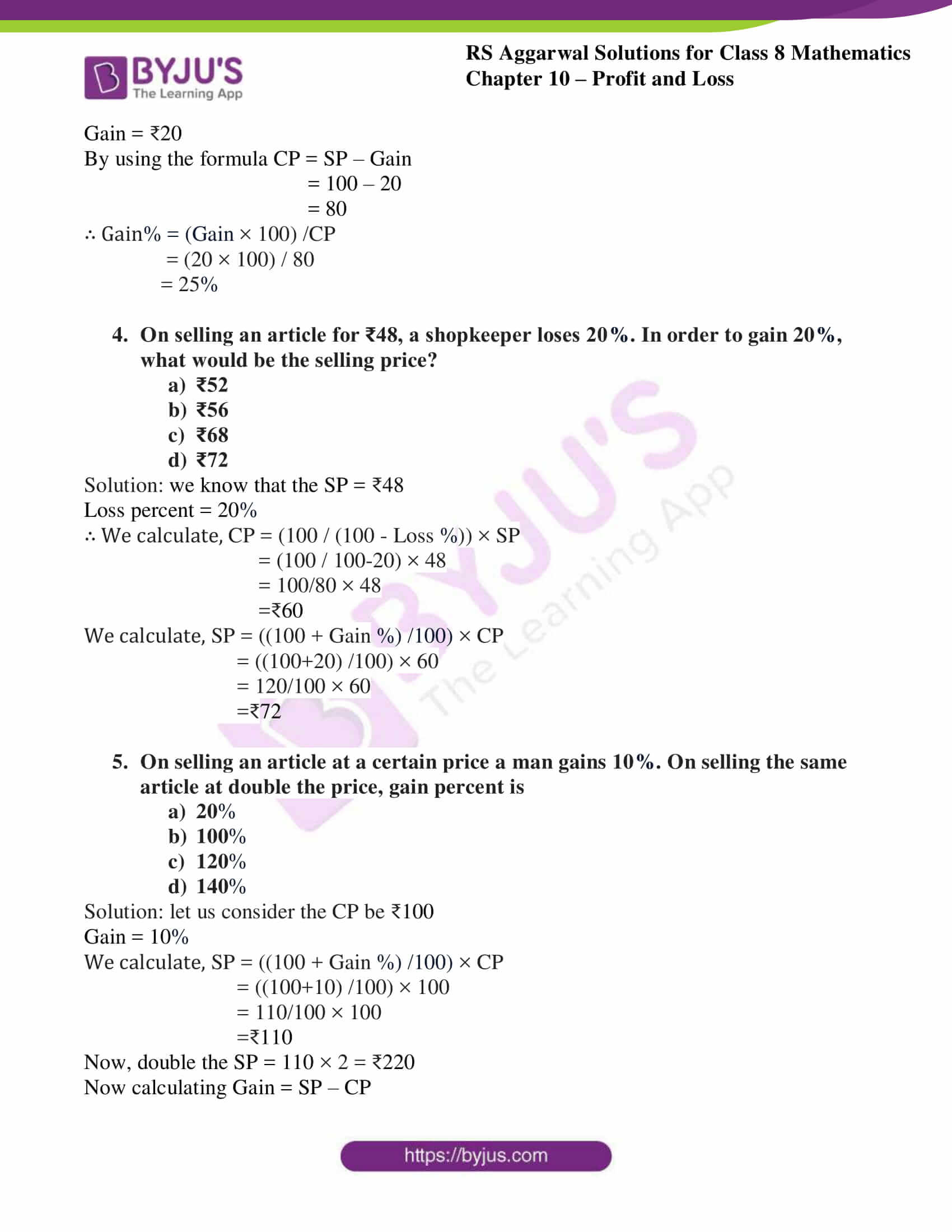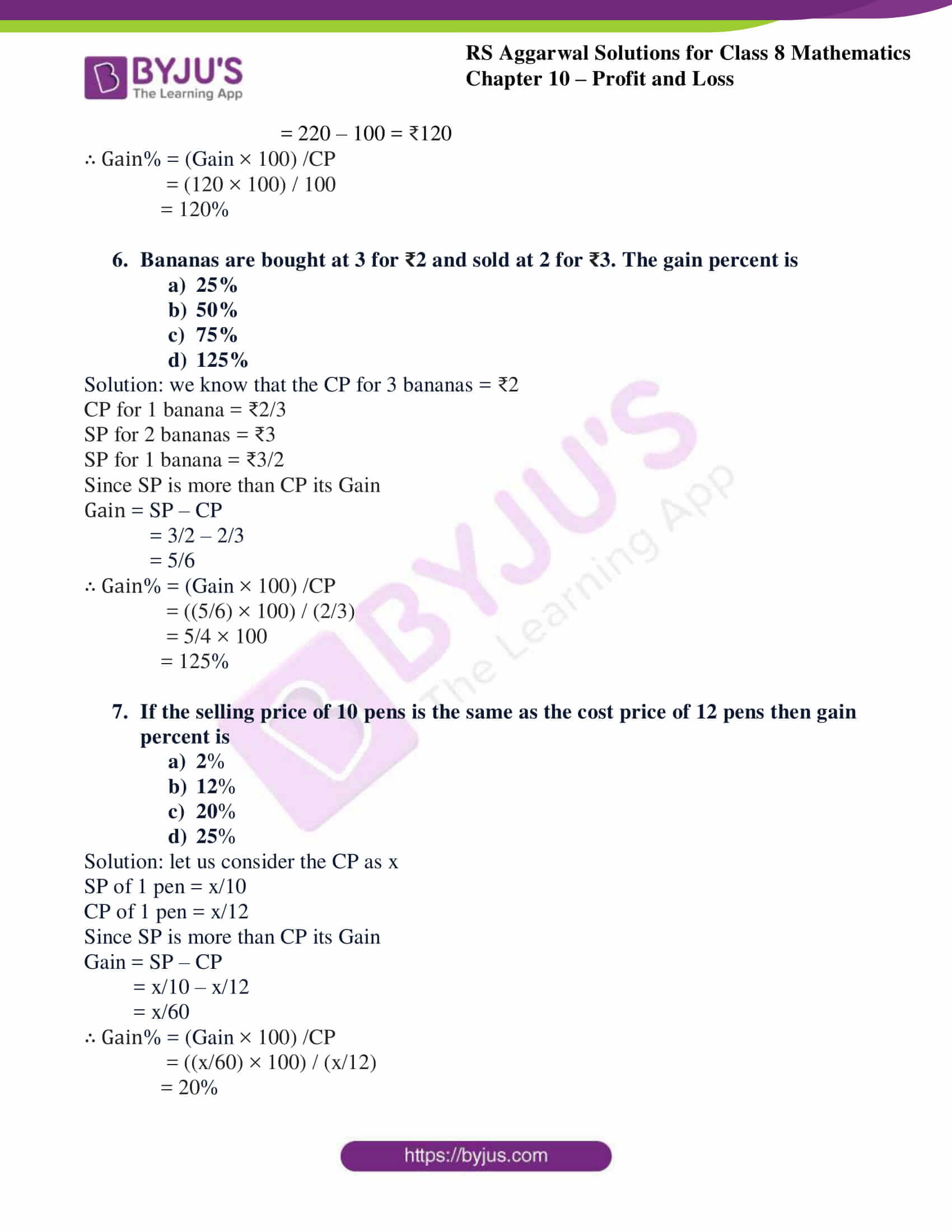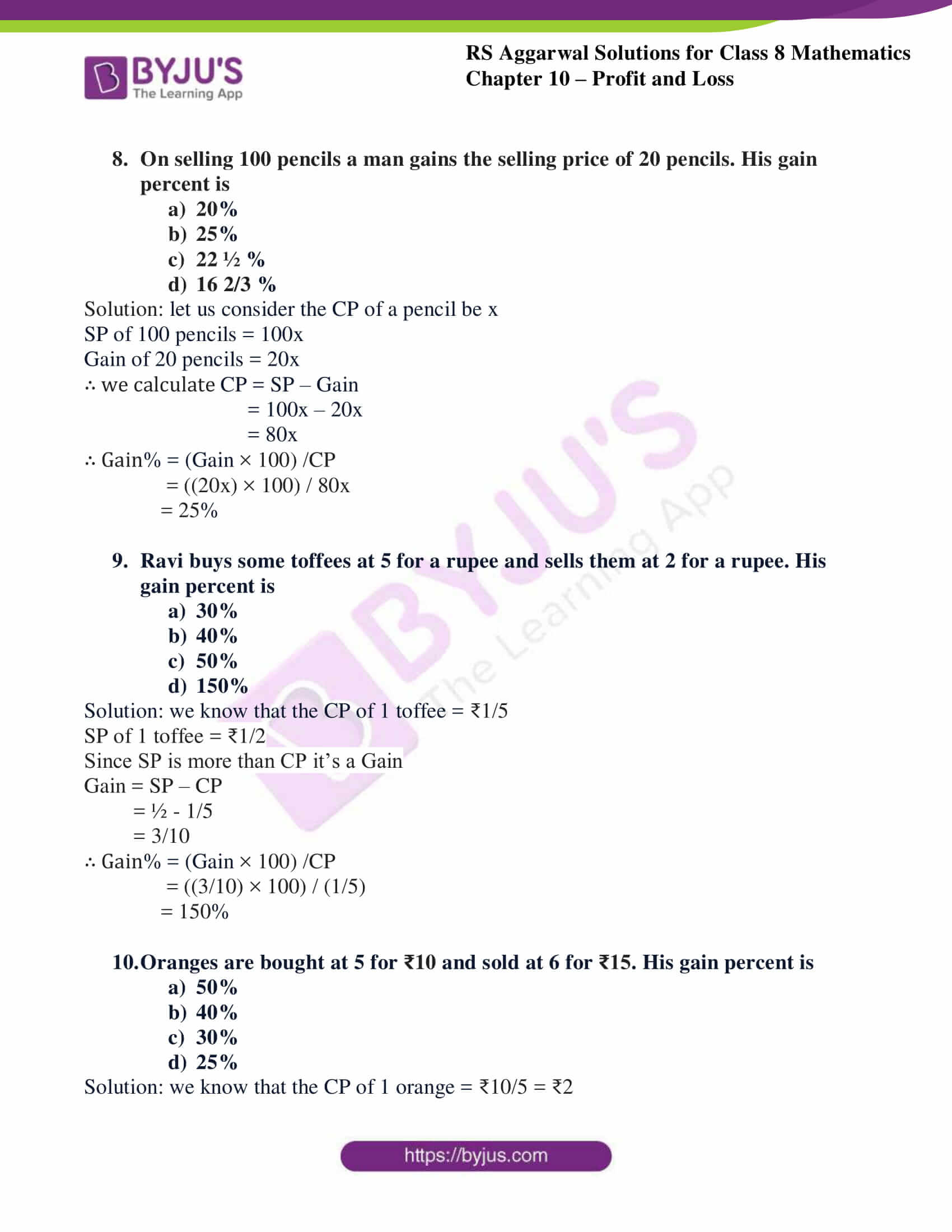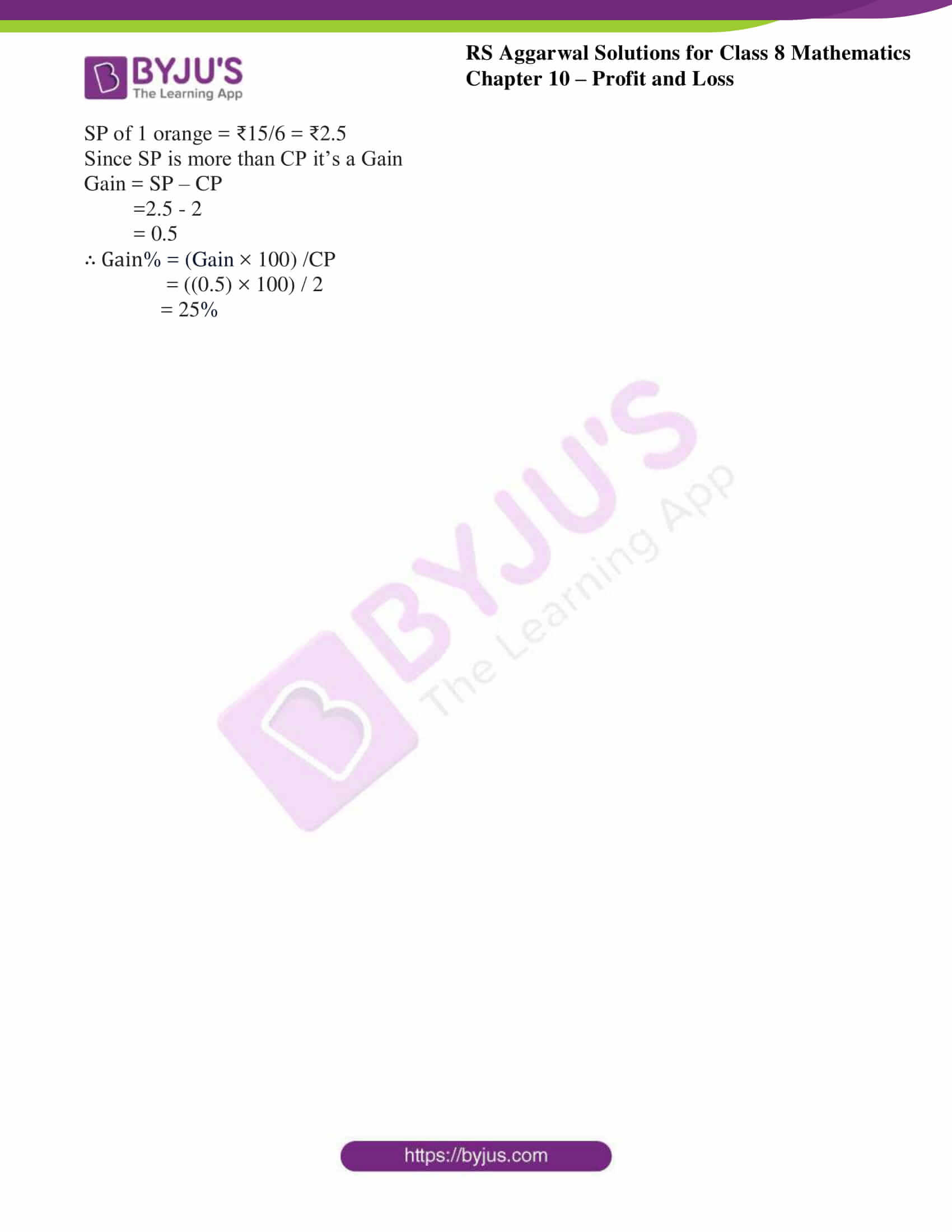### Access Answers to RS Aggarwal Solutions for Class 8 Maths Chapter 10- Profit and Loss Exercise 10D

Select the correct answer in each of the following:

1. Rajan buys a toy for ₹75 and sells it for ₹100. His gain percent is

1. 25%
2. 20%
3. $33\frac{1}{3}$%
4. $37\frac{1}{2}$%

Solution: we know that the CP = 75

SP = 100

Since SP is more than CP it’s a gain

Gain = SP – CP

= 100 – 75

= 25

∴ Gain% = (Gain × 100) /CP

= (25 × 100) / 75

= 33.33%

2. A bat is bought for ₹120 and sold for ₹105. The loss percent is

1. 15%
2. 12 ½%
3. $16\frac{2}{3}$%
4. $14\frac{1}{5}$%

Solution: we know that the CP = ₹120

SP = ₹105

Since SP is less than CP it’s a loss

Loss = CP – SP

= 120 – 105

= 15

∴ Loss% = (loss × 100) /CP

= (15 × 100) / 120

= 12.5%

3. A bookseller sells a book for ₹100, gaining ₹20. His gain percent is

1. 20%
2. 25%
3. 22%
4. None of these

Solution: we know that the SP = ₹100

Gain = ₹20

By using the formula CP = SP – Gain

= 100 – 20

= 80

∴ Gain% = (Gain × 100) /CP

= (20 × 100) / 80

= 25%

4. On selling an article for ₹48, a shopkeeper loses 20%. In order to gain 20%, what would be the selling price?

1. ₹52
2. ₹56
3. ₹68
4. ₹72

Solution: we know that the SP = ₹48

Loss percent = 20%

∴ We calculate, CP = (100 / (100 – Loss %)) × SP

= (100 / 100-20) × 48

= 100/80 × 48

=₹60

We calculate, SP = ((100 + Gain %) /100) × CP

= ((100+20) /100) × 60

= 120/100 × 60

=₹72

5. On selling an article at a certain price a man gains 10%. On selling the same article at double the price, gain percent is

1. 20%
2. 100%
3. 120%
4. 140%

Solution: let us consider the CP be ₹100

Gain = 10%

We calculate, SP = ((100 + Gain %) /100) × CP

= ((100+10) /100) × 100

= 110/100 × 100

=₹110

Now, double the SP = 110 × 2 = ₹220

Now calculating Gain = SP – CP

= 220 – 100 = ₹120

∴ Gain% = (Gain × 100) /CP

= (120 × 100) / 100

= 120%

6. Bananas are bought at 3 for ₹2 and sold at 2 for ₹3. The gain percent is

1. 25%
2. 50%
3. 75%
4. 125%

Solution: we know that the CP for 3 bananas = ₹2

CP for 1 banana = ₹2/3

SP for 2 bananas = ₹3

SP for 1 banana = ₹3/2

Since SP is more than CP its Gain

Gain = SP – CP

= 3/2 – 2/3

= 5/6

∴ Gain% = (Gain × 100) /CP

= ((5/6) × 100) / (2/3)

= 5/4 × 100

= 125%

7. If the selling price of 10 pens is the same as the cost price of 12 pens then gain percent is

1. 2%
2. 12%
3. 20%
4. 25%

Solution: let us consider the CP as x

SP of 1 pen = x/10

CP of 1 pen = x/12

Since SP is more than CP its Gain

Gain = SP – CP

= x/10 – x/12

= x/60

∴ Gain% = (Gain × 100) /CP

= ((x/60) × 100) / (x/12)

= 20%

8. On selling 100 pencils a man gains the selling price of 20 pencils. His gain percent is

1. 20%
2. 25%
3. 22 ½ %
4. 16 2/3 %

Solution: let us consider the CP of a pencil be x

SP of 100 pencils = 100x

Gain of 20 pencils = 20x

∴ we calculate CP = SP – Gain

= 100x – 20x

= 80x

∴ Gain% = (Gain × 100) /CP

= ((20x) × 100) / 80x

= 25%

9. Ravi buys some toffees at 5 for a rupee and sells them at 2 for a rupee. His gain percent is

1. 30%
2. 40%
3. 50%
4. 150%

Solution: we know that the CP of 1 toffee = ₹1/5

SP of 1 toffee = ₹1/2

Since SP is more than CP it’s a Gain

Gain = SP – CP

= ½ – 1/5

= 3/10

∴ Gain% = (Gain × 100) /CP

= ((3/10) × 100) / (1/5)

= 150%

10. Oranges are bought at 5 for ₹10 and sold at 6 for ₹15. His gain percent is

1. 50%
2. 40%
3. 30%
4. 25%

Solution: we know that the CP of 1 orange = ₹10/5 = ₹2

SP of 1 orange = ₹15/6 = ₹2.5

Since SP is more than CP it’s a Gain

Gain = SP – CP

=2.5 – 2

= 0.5

∴ Gain% = (Gain × 100) /CP

= ((0.5) × 100) / 2

= 25%

### Access other Exercises of RS Aggarwal Solutions for Class 8 Maths Chapter 10- Profit and Loss

Exercise 10A Solutions 34 Questions

Exercise 10B Solutions 14Questions

Exercise 10C Solutions 11 Questions

## RS Aggarwal Solutions for Class 8 Maths Chapter 10- Profit and Loss Exercise 10D

Exercise 10D of RS Aggarwal Class 8, Profit and Loss. This exercise mainly deals with the Objective Questions related to the topics discussed earlier in Chapter 10. The RS Aggarwal Solutions which provides step-wise solutions ensures that students get to know the best approach to the individual questions. By referring to these solutions, students develop problem-solving abilities and can tackle any type of questions easily.

Students are suggested to practice the problems on a regular basis which will help them excel in their exams and increase their overall percentage. Practicing as many times as possible helps in building time management skills and also boosts the confidence level to achieve high marks.